# Coordination Chemistry

## 20 Questions MCQ Test GATE Chemistry Mock Test Series | Coordination Chemistry

Description
Attempt Coordination Chemistry | 20 questions in 60 minutes | Mock test for Chemistry preparation | Free important questions MCQ to study GATE Chemistry Mock Test Series for Chemistry Exam | Download free PDF with solutions
QUESTION: 1

### A complex of Ni(ll), [NiCl2(PPh3)2] is paramagnetic, the analogous complex of Palladium (II) is diamagnetic, the number of isomers shown by both complexes respectively are

Solution: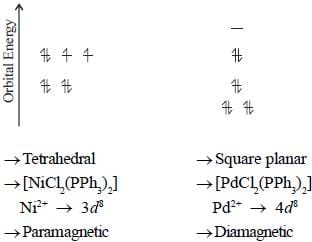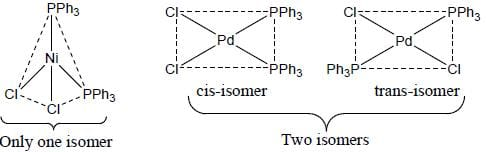QUESTION: 2

### The spin-only magnetic moment and the spectroscopic ground state term symbol of iron centre in FeO69- ion respectively are

Solution: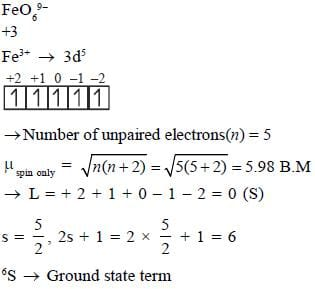QUESTION: 3

### Among the following metal complexes in order of their increasing hydration energy.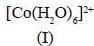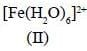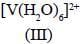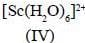Solution:

Hydration energy is released when gaseous metal cations get hydrated.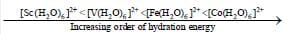QUESTION: 4

The true statement about base hydrolysis of [Co(CN)5JsBr]3- is

Solution:

The base hydrolysis of Co(lll) complexes with ligand without replaceable proton like [Co(CN)5Br]3- was found to be very low and independent of the concentration of nucleophile. Since the non-leaving ligand do not contain replaceable proton, the SN1CB mechanism is not possible.

QUESTION: 5

In square planar complexes which of the d -orbital / orbitals get direct interaction with ligand orbitals

Solution:

In square planar complexes, dx-yorbital get direct interaction with ligand orbital.

QUESTION: 6

The Δ0 value of [Co(NH3)6]3+ is 22,870 cm -1. The Δ0 value of [CoCH2O6]3+ and [C oF6]3- - compared to [Co(NH3)6]3+ respectively are

Solution: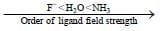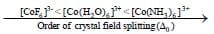*Answer can only contain numeric values
QUESTION: 7

The total number of micro states for 3F, 3P and 1D is____________

Solution: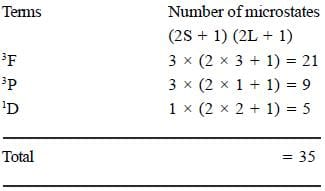*Answer can only contain numeric values
QUESTION: 8

The oxidation state of Ru metal ion in [Ru(Terpy)2)J (PF6)2 is__________

Solution: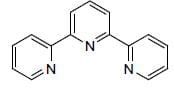Terpyndine (neutral ligand)
or
Terpy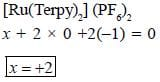*Answer can only contain numeric values
QUESTION: 9

Potassium ferrocyanide reacts with nitric acid to form product A. Which further reacts with sodium carbonate to form product B. The total number of elections in t2g orbitals of metalion present in product B is

Solution: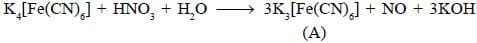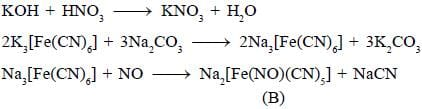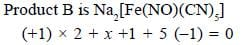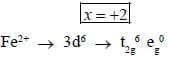*Answer can only contain numeric values
QUESTION: 10

Among the following ligands, the number of ligands that can not act as ambidentate ligands is_________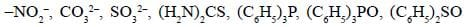Solution:

The monodentate ligands which have two or more different donor atoms can coordinate to a metal cation through either of the two different donor sites. These ligands are called ambidentate ligands.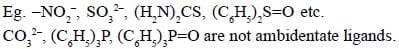QUESTION: 11

Among the following complexes, the correct trend of Pt-N bond strength is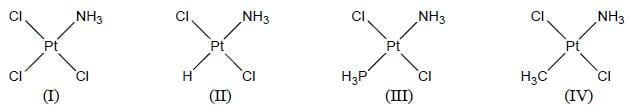Solution:

The trans-Pt-N bond is weakened as T becomes a better labilizer of the trans-ammonia and stabilization of the transition state must also be an important contribution to the trans-effect. The strength of P t- N bond in trans-[PtCl2(NH3)T] decreases as T is changed in the order

QUESTION: 12

The correct trend of rate of electron transfer reaction between the complexes [CoC NH3),5X]2+/ [Co(H2O)6]2+ with variation of X is

Solution:

The electron transfer between complexes [Co(NH3,)5X]2+ / [Co(H2O6 )2+ is a type o f inner sphere mechanism.
The typical bridging ligands involved in such reaction are capable of conveying the electron and contain more than one lone pair of electrons.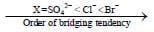QUESTION: 13

The spmel structure of Cr2FeO4 has cubic close packed arrangement of oxide ions. The fractions of the tetrahedral and octahedral sites occupied by cation respectively are

Solution: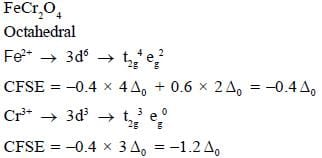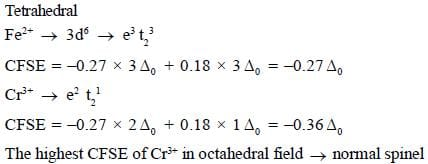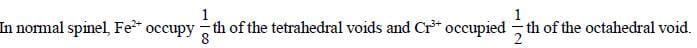QUESTION: 14

The blue colour of [CoCl4]2- is due to the transition of

Solution:

In tetrahedral [CoCI4]2-  ion the visible region transition is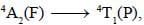which is responsible for blue colour.

QUESTION: 15

Which of the following octahedral complex exhibits tetragonal compression

Solution:

In the crystalline of KCuF3, the two Cu-F distance on z-axis are 1.96Å and the remaining four Cu-F distance in xy-plane are at 2.07Å. It is due to Jahn-Teller compression.

QUESTION: 16

The colour and tlie mason for the deep coloration of [Fe(NCS)6]3- and [Fe(bpy)3]2+ respectively are

Solution: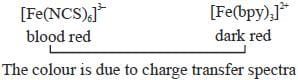*Answer can only contain numeric values
QUESTION: 17

The total number of isomers possible for the molecule [Co(en)2Cl(NO2)]+ is___________

Solution: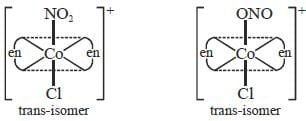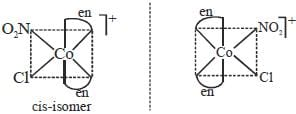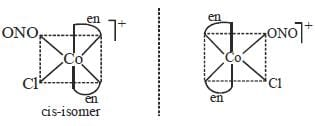Total number of isomer is six

*Answer can only contain numeric values
QUESTION: 18

The total sum of x value of the following complexes is __________________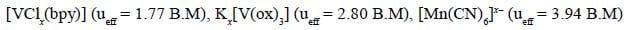Solution: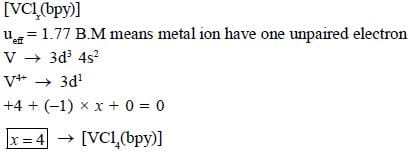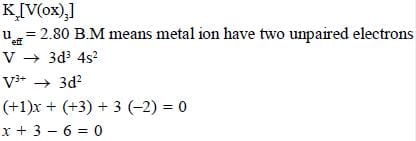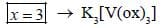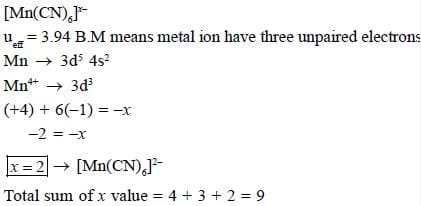*Answer can only contain numeric values
QUESTION: 19

A complex [Co(h2O)6]2+ has an absorption maxima at 510 nm. The crystal field stabilisation energy for [CoC14]2- (in KJ mol-1) is _______________(Ignoring stabilisation charge)

Solution: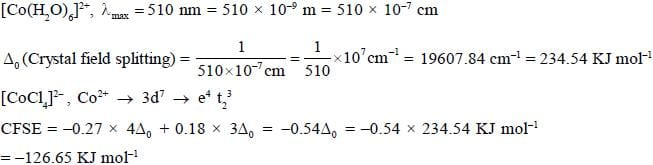*Answer can only contain numeric values
QUESTION: 20

In [Ni(PNP)X]2 [NiX4] complex, the total coordination number of metal ion is______________
(X is a uninegative monodentate ligand)

Solution:

The compound [Ni(PNP)X]2 [NiX4] where PNP (tridentate) =
(C6H5)2PCH2CH2NRCH2CH2P(C6H5)2 the total coordination number of metal ion is 12.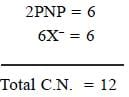Use Code STAYHOME200 and get INR 200 additional OFF Use Coupon Code